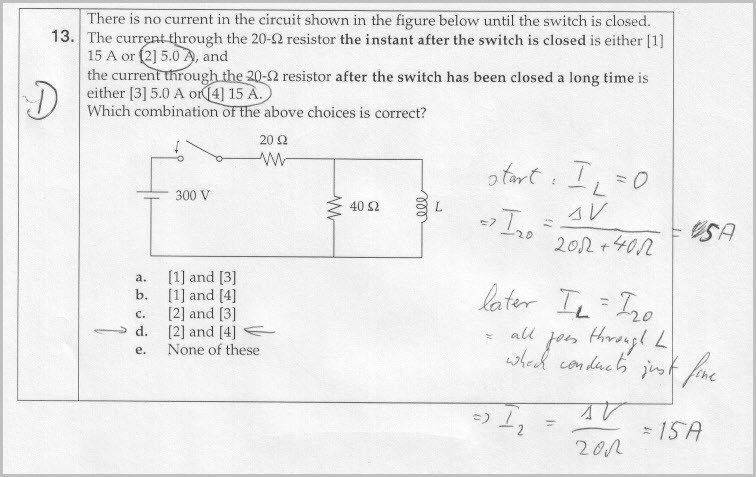# How the current go through this circuit?

• machinarium

## Homework Statement## The Attempt at a Solution

I don't understand why at the start, I20 was calculated like that, and why for a long time, IL=I20

Does that mean for the instant the switch was closed, the current just went through the resistors? And for a long time, the current skipped 40 ohm resistor? Why was that?

I think this is a basic knowledge but I can't remember what it is.

#### Attachments

I don't understand why at the start, I20 was calculated like that, and why for a long time, IL=I20

Does that mean for the instant the switch was closed, the current just went through the resistors?

Yes.

And for a long time, the current skipped 40 ohm resistor? Why was that?

Yes.

I think this is a basic knowledge but I can't remember what it is.

Inductors "oppose" rapid changes in current through them. It's analogous to inertia. They produce what's called a back-emf, a voltage that opposes the attempted change in current.

V = L*dI/dt

Once the current stops changing, the back-emf goes to zero. At that point the effective resistance (impedance) of the inductor is zero. Note that this only applies to a constant current situation.

So the rule of thumb for inductors in DC circuits is, they look like open circuits when voltage is first applied (usually at t=0), and like short circuits after a long time ("a long time" can be taken to be more than about 5 time constants for the circuit).

For capacitors the opposite holds. They tend to oppose changes in voltage across them, so they look like short circuits at start-up, and when they settle down to their full charge in the (DC) circuit, they look like open circuits.

I understand it. Thank you very much, gneill.